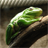StaffJoined:

May 27, 2014

## JSL-Unit: Automated JSL Testing

Unit testing for JSL applications may be accomplished with the JSL-Unit GUI driver script (i.e. RunJSL_Unit.jsl) and a set of unit test scripts and tables. The unit test scripts and tables are those JSL and JMP files in the tests directory (or one of its subdirectories) with the prefix test (e.g. test geometric mean.jsl). The driver defines a ut assert function, which each unit test should call for each test case. The prototype for ut assert is:

ut assert(expression, expectedvalue <,stresscase="N">)

The first argument is an expression which, when evaluated, evaluates to the actual value of the test case and the second argument is the expected value of the test case. Note that expression and expectedvalue are required arguments but stresscase is optional, with a default value of N. The stresscase argument is intended for those test cases that may be considered rare events and are likely to result in a failure and so may be bypassed for typical test runs.
Note: The GUI driver provides a checkbox that, when checked, includes stress test cases.

Numeric test results are compared using an epsilon to represent the acceptable variation (i.e. fuzzy comparison). There are two epsilon values, an absolute epsilon is used when the expected result is 0 and a relative epsilon is used for all other cases. The epsilon values are reset to the default values before running each test script, and these are global variables that a test script can overwrite if desired.

ut zero epsilon = 1e-12;  // close enough when expected is 0
ut relative epsilon = 1e-9;  // close enough relative to expected value

Run details are written to the log. For failures, the actual and the expected result, plus the LRE (for numeric tests only) are reported. LRE is an acronym that refers to the Logarithm (base 10) of the Relative Error (i.e. -log10(abs(q - c)/abs(c)).

Note: There is also a ut assert variant called ut assert digits for when you want to set epsilon on the fly to test for a specified number of digits of accuracy. It adds a third argument which is the number of digits of accuracy required. This is especially useful when the expected value is only provided to a certain number of digits. For example,

ut assert digits(Expr(Sqrt(2)), 1.414, 4);  // is sqrt(2) accurate to 4 digits
ut assert digits(Expr(Pi()), 3.14159, 6);  // is pi() accurate to 6 digits

ut assert digits also provides the stress case argument as a fourth, optional, argument. For test data tables, epsilon values should be stored as table variables and used in computing the pass/fail result.